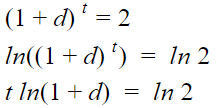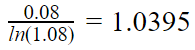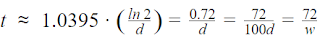# The Rule of 72: how long it will take to double your investment

How do seasoned investors know how to make lightning-quick decisions? What kind of magic tricks do they have up their sleeve?

A lot of this comes from experience, but there are some handy shortcuts that any burgeoning investor can consider. One of these shortcuts is the Rule of 72.

This rule is a reasonably accurate estimate of how long an investment is going to take to double with a fixed annual rate of interest. All you have to do is divide the number 72 by the annual rate of return to obtain a rough estimate of the number of years it will take for your initial investment to double.

### Calculating Using The Rule of 72

For example, if an investment scheme that you’ve been eyeing promises around an annual growth rate of 6%, then 72/6 = 12. This means you’ll be getting double your original investment back in twelve years.

It’s a super useful shortcut you can use on the go to get a rough idea of how long you have to wait to see double the returns.

For clarity, you’ll be using the annual return as it is, and not in decimal form. This is so you get the result in years.

### Other Ways To Use The Rule of 72

You can also work this rule backward to find different values. To demonstrate, say you’re hoping to double your money in 5 years. Divide 72 by 5 and the answer (14.4) is how much annual growth rate you’ll need to double your principal in five years.

If it grows, it goes. The Rule of 72 can be used to estimate inflation, interest, and even population.

For example, it’s been found that your country’s GDP grows at 3% a year. This means that the economy is expected to double in 24 years (72/3 = 24). If this percentage gets pushed up by even one percent, then the economy doubles in 18 years (72/4 = 18).

On a personal scale, this principle can also be used on credit card fees. If you sign up for a credit card with 12% interest, then the amount you owe the company will double in six years (so pay your card off in time!).

### Where did this come from?

This rule is derived as follows. Assume the following:
`````` t = Time to double
ln = natural log function
d = compounded interest rate per period in decimal number
w = 100d so interest rate in whole numbers```
```

If d = 0.06 and t = 12, then (1+0.06)12 = 2.012

In other words, if you compound 6% interest twelve times, you arrive just above doubling the original principal, just like the Rule of 72 would suggest.

If we move things around a bit, we get the following (remember logarithmic rules?):That's nice. If we then divide by t, later add d to numerator and denominator and move things around a little, we get:This is quite helpful, since the ln (1+d) stays close to just d with small d values and thus, the right parenthesis stays close to 1.

If we take an appropriate example for d, e.g. 8%, we can simplify our calculations, while still keeping it quite precise.

The right parenthesis becomes an approximate constant:We then use this approximation for the righter parenthesis. Multiplying with ln 2 we get approximately 0.72.

We then multiply both the numerator and denominator by 100 to get to whole numbers.Ta-da! There's the Rule of 72!

To find out how long it would take to double an investment with 8% returns annually, you would calculate it like this:

T = ln(2) / ln(1+0.08)) = 9.006 years

This value is close to the approximate value obtained by the Rule of 72 (72 / 8) = 9 years. Because logarithmic calculations can be a real chore and require the use of scientific calculators, this was eventually simplified to The Rule.

### Limitations Of The Rule

As long as the interest rate is less than 20%, this tool is an incredibly accurate measure for returns. Any more than that and the likelihoods of error start to become significant. At 20% a more accurate number to use would be 76 and at just 2% the number would be 70.

But, 72 is both good in the right range, plus it can be divided with 2, 3, 4, 6, 8, 9, 12 and 18.

While it can be helpful for helping you gain a quick understanding of the value of an investment, it should not be used on its own. Remember to conduct additional research and consult any investment professionals to help you make better, more informed decisions.

Happy investing!
Blog posts may contain affiliate links

• 3 weeks ago
• 7 months ago
• 2 years ago